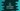# C# program to print the area and perimeter of a square## C# program to print the area and perimeter of a square:

In this post, we will learn how to print the area and perimeter of a square. The program will take the size of one side of the square as input from the user and calculate its perimeter and area.

### How to find the area and perimeter of a square:

If we multiply the length of one side with another side, it will give the area and if we add all sides, it will give the perimeter of a square.

Since the length of all sides of a square is equal, the area will be square of one side and the perimeter will be 4 * size of one side.

### Algorithm to calculate the area and perimeter of a square:

We can use the below algorithm to calculate the area and perimeter of a square:

• Take the length of one side of the square as input from the user. Store it in a variable.
• Calculate the area of the square by calculating the square of the side and perimeter by multiplying the side by 4.
• Print the calculated area and perimeter values to the user.

### Example 1: C# program to print the area and perimeter of a square with predefined side:

The below program calculates and prints the area and perimeter of a square with predefined side size:

``````namespace Program
{

class Program
{
static void Main(string[] args)
{
decimal Side = 10;

decimal Area = Side * Side;
decimal Perimeter = 4 * Side;

Console.WriteLine("Square side: " + Side);
Console.WriteLine("Area of the square: " + Area);
Console.WriteLine("Perimeter of the square: " + Perimeter);
}
}
}``````

Here, 10 is the side of the square. The calculated area and perimeter are stored in the variables Area and Perimeter.

If you run this program, it will print the below output:

``````Square side: 10
Area of the square: 100
Perimeter of the square: 40``````

### Example 2: C# program to print the area and perimeter of a square with user given size:

We can also take the size of the square as an input from the user and calculate the area and perimeter. For example:

``````namespace Program
{

class Program
{
static void Main(string[] args)
{
decimal Side;

Console.WriteLine("Enter the size of the side: ");

decimal Area = Side * Side;
decimal Perimeter = 4 * Side;

Console.WriteLine("Square side: " + Side);
Console.WriteLine("Area of the square: " + Area);
Console.WriteLine("Perimeter of the square: " + Perimeter);
}
}
}``````

It is taking the side of the square as input from the user and prints the area and perimeter.

It will give similar result.

``````Enter the size of the side:
10
Square side: 10
Area of the square: 100
Perimeter of the square: 40``````

### Example 3: C# program to print the area and perimeter of a square with user given size and separate function:

Let’s write a separate function to find the area and perimeter of the square. The Main method will pass the side length to the function and it will calculate and print the area and perimeter values.

``````namespace Program
{

class Program
{
static void PrintAreaPerimeter(decimal side)
{
decimal Area = side * side;
decimal Perimeter = 4 * side;

Console.WriteLine("Square side: " + side);
Console.WriteLine("Area of the square: " + Area);
Console.WriteLine("Perimeter of the square: " + Perimeter);
}
static void Main(string[] args)
{
decimal Side;

Console.WriteLine("Enter the size of the side: ");

PrintAreaPerimeter(Side);
}
}
}``````

In this program, a new function PrintAreaPerimeter is used to calculate and print the area and perimeter of a square. It takes the side length as its parameter and prints the calculated values.

If you run this program, it will give similar result:

``````Enter the size of the side:
12
Square side: 12
Area of the square: 144
Perimeter of the square: 48``````